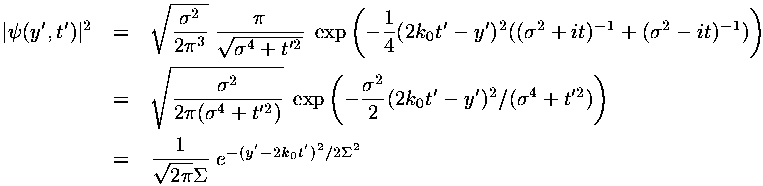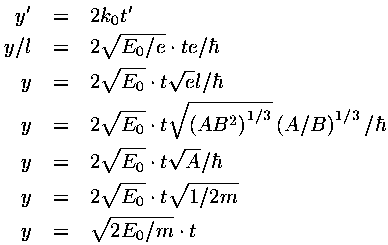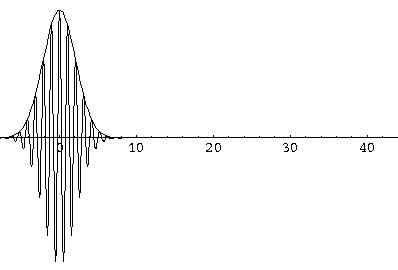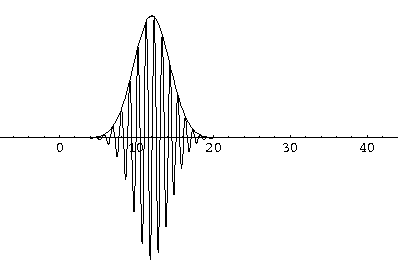## Falling p.12

### Falling in 2 Dimensions: Visualizing y Solutions

We have found a particular solution to the time dependent Schrödinger's equation:This solution represents a superposition of single-energy solutions making a lump of probability that movesIn dimensionless units the velocity of the wave-packet is 2k0. If we convert this to normal quantities we find:i.e., the wave-packet moves with the velocity expected for a particle with that (average) energy.

In the above the primed quantities are dimensionless versions of the usual variables (e.g., y vs y'). I'll now drop the primes, but everything is to be considered the dimensionless quantity.

There is lots to be learned by carefully examining graphs of this solution. It is hard, however, to plotin the usual way on the "y" axis, sincetakes on complex (rather than just real) values. My solution here is to plot both the real part of(which shows oscillations) and the absolute value of. You can estimate the imaginary part ofby looking at the difference between || and Re().

The variable k0 determines the typical "wavelength" of the oscillations:

k0 = 2/so short wavelength corresponds to large k0. In the below, note that when k0=6 the wavelength is about 1, and when k0=3 the wavelength is about 2. Recall that the fourier transform g(k) shows which simple waves make up the wave-packet. Thus the peak of g(k) is at k0.The variabledetermines the initial width of the lump of probability. At the same time (because of Heisenberg's Uncertainty) it determines the range of speeds (momentums, energies) that contribute significantly to the superposition. Large spatial extent means a reduced range of wavelengths to make up the packet. Thus while the peak of g(k) is at k0, the width of the peak is determined by. Large-sized wave-packets have a largeand a narrow peak in g(k). (If the spatial extent of the wavefunction is large the waves that make up the packet must have quite similar wavelengths, otherwise those waves could not be in-phase within the packet, which is required for constructive interference.)Here are some pictures of a k0=6,=1.5 wavefunction at various times.

### t=0### t=1### t=2### t=3Notice that the central part of the wave packet moved from y=0,12,24,36 as t=0,1,2,3, that is to say that the "velocity" of the packet is about 12=2k0. Also notice that, as expected, the packet has expanded slightly.

Notice that within the lump of probability you can see the waves of the real part of. In the following sequence of stills (for t=0, 0.1, 0.2, 0.3, ...), we follow one of these peaks in Re() within the wave-packet. Notice that this particular peak (marked in red) falls behind the wave-packet. During the 1/2 tic time period displayed, the wave-packet as a whole has moved forward 6 units, whereas the individual peak has moved forward 3 units. The speed of an individual peak is called the phase velocity; the speed of the wave-packet is called the group velocity. For a free particle in QM the phase velocity is half the group velocity.All of these features can be seen in greater detail by examining the following QuickTime movie (4 Mb) on a frame-by-frame basis.

It is also interesting to view the moving wave in a frame that moves with the wave, as in this QuickTime movie (4 Mb).

Finally, I have stated that we can attribute the spreading of the wave-packet to the different velocities that make up the packet. Classically you should expect that the faster aspects of the wave-packet should eventually move ahead of the slower. Thus since de Broglie's relationship associates the local wavelength with the particle's momentum, we might expect that the local wavelength at the front of the packet becomes shorter than the local wavelength at the rear of the wave-packet. In our examples we have chosento minimize the velocity differences, so the effect is small. Nevertheless, careful examination of this t'=5 frame shows that the local wavelength at the front of this wave is smaller than the wavelength at the rear of the wave: